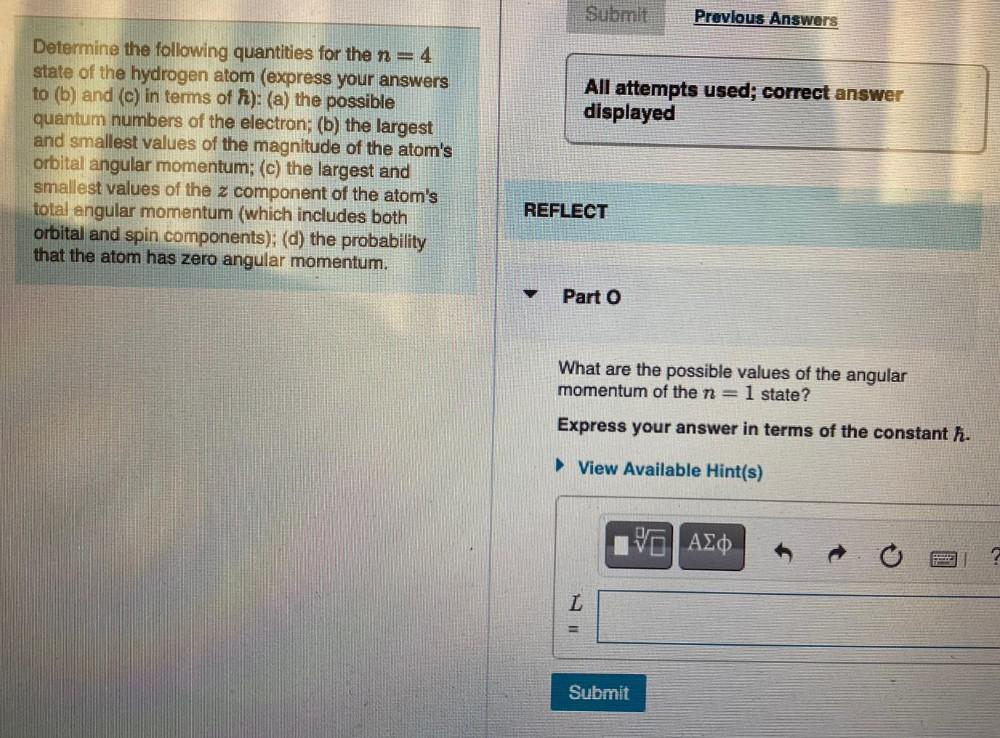Question:

# Submit Previous Answers All attempts used; correct answer displayed Determine the following quantities for the n=4 state of theSubmit Previous Answers All attempts used; correct answer displayed Determine the following quantities for the n=4 state of the hydrogen atom (express your answers to (b) and (c) in terms of f): (a) the possible quantum numbers of the electron; (b) the largest and smallest values of the magnitude of the atom's orbital angular momentum; (c) the largest and smallest values of the z component of the atom's total angular momentum (which includes both orbital and spin components); (d) the probability that the atom has zero angular momentum. REFLECT Part 0 What are the possible values of the angular momentum of the n=1 state? Express your answer in terms of the constant h. ► View Available Hint(s) VALO L Submit